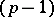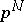##### Actions

(diff) ← Older revision | Latest revision (diff) | Newer revision → (diff)
C. Rader [a2] observed that a Fourier transform computation of prime sizecould be computed by a-point cyclic convolution. S. Winograd [a4] extended Rader's result to Fourier transform computations of prime power sizeand introduced cyclic convolution algorithms based on the polynomial version of the Chinese remainder theorem to compute the resulting cyclic convolutions (cf. Winograd small convolution algorithm). The overall strategy is usually called the Winograd fast Fourier transform algorithm, or Winograd FFT algorithm. Rader computed the-point cyclic convolution by calling on the convolution theorem to turn the-point convolution into several-point Fourier transform computations.